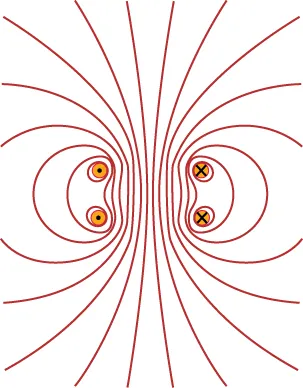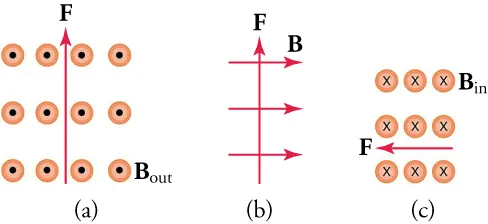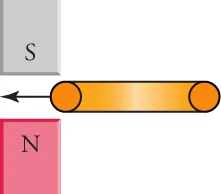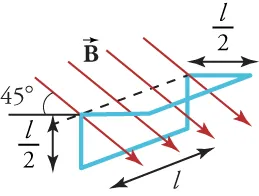Physics

# Extended Response

PhysicsExtended Response

### Extended Response

#### 20.1Magnetic Fields, Field Lines, and Force

49.

Summarize the properties of magnets.

1. A magnet can attract metals like iron, nickel, etc., but cannot attract nonmetals like piece of plastic or wood, etc. If free to rotate, an elongated magnet will orient itself so that its north pole will face the magnetic south pole of Earth.
2. A magnet can attract metals like iron, nickel, etc., but cannot attract nonmetals like piece of plastic or wood, etc. If free to rotate, an elongated magnet will orient itself so that its north pole will face the magnetic north pole of Earth.
3. A magnet can attract metals like iron, nickel, etc., and nonmetals like piece of plastic or wood, etc. If free to rotate, an elongated magnet will orient itself so that its north pole will face the magnetic south pole of Earth.
4. A magnet can attract metals like iron, nickel, etc., and nonmetals like piece of plastic or wood, etc. If free to rotate, an elongated magnet will orient itself so that its north pole will face the magnetic north pole of Earth.
50.

The magnetic field shown in the figure is formed by current flowing in two rings that intersect the page at the dots. Current flows into the page at the dots with crosses (right side) and out of the page at the dots with points (left side).Where is the field strength the greatest and in what direction do the magnetic field lines point?

1. The magnetic field strength is greatest where the magnetic field lines are less dense; magnetic field lines points up the page.
2. The magnetic field strength is greatest where the magnetic field lines are most dense; magnetic field lines points up the page.
3. The magnetic field strength is greatest where the magnetic field lines are most dense; magnetic field lines points down the page.
4. The magnetic field strength is greatest where the magnetic field lines are less dense; magnetic field lines points down the page.
51.

The forces shown below are exerted on an electron as it moves through the magnetic field. In each case, what direction does the electron move?1. (a) left to right, (b) out of the page, (c) upwards
2. (a) left to right, (b) into the page, (c) downwards
3. (a) right to left, (b) out of the page, (c) upwards
4. (a) right to left, (b) into the page, (c) downwards

#### 20.2Motors, Generators, and Transformers

52 .
Explain why increasing the frequency of rotation of the coils in an electrical generator increases the output emf.
1. The induced emf is proportional to the rate of change of magnetic flux with respect to distance.
2. The induced emf is inversely proportional to the rate of change of magnetic flux with respect to distance.
3. The induced emf is inversely proportional to the rate of change of magnetic flux with respect to time.
4. The induced emf is proportional to the rate of change of magnetic flux with respect to time.
53.

Your friend tells you that power lines must carry a maximum current because P = I2R, where R is the resistance of the transmission line. What do you tell her?

1. Ptransmitted = Itransmitted2Rwire and Plost = Itransmitted Vtransmitted, so I must be high to reduce power lost due to transmission.
2. Plost = Itransmitted2Rwire and Plost = Itransmitted Vtransmitted, so I must be high to reduce power lost due to transmission.
3. Ptransmitted = Itransmitted2Rwire and Plost = Itransmitted Vtransmitted, so I must be low to reduce power lost due to transmission.
4. Plost = Itransmitted2Rwire and Plost = Itransmitted Vtransmitted, so I must be low to reduce power lost due to transmission.

#### 20.3Electromagnetic Induction

54.

When you insert a copper ring between the poles of two bar magnets as shown in the figure, do the magnets exert an attractive or repulsive force on the ring? Explain your reasoning.1. Magnets exert an attractive force, because magnetic field due to induced current is repulsed by the magnetic field of the magnets.
2. Magnets exert an attractive force, because magnetic field due to induced current is attracted by the magnetic field of the magnets.
3. Magnets exert a repulsive force, because magnetic field due to induced current is repulsed by the magnetic field of the magnets.
4. Magnets exert a repulsive force, because magnetic field due to induced current is attracted by the magnetic field of the magnets.
55.

The figure shows a uniform magnetic field passing through a closed wire circuit. The wire circuit rotates at an angular frequency of about the axis shown by the dotted line in the figure.What is an expression for the magnetic flux through the circuit as a function of time?

1. expression for the magnetic flux through the circuit Φ(t) = BAcos⁡ωt
2. expression for the magnetic flux through the circuit $Φ(t)= 2 BAcosωt Φ(t)= 2 BAcosωt$
3. expression for the magnetic flux through the circuit $Φ(t)= 3 BAcosωt Φ(t)= 3 BAcosωt$
4. expression for the magnetic flux through the circuit Φ(t) = 2BA cos ωt
Order a print copy

As an Amazon Associate we earn from qualifying purchases.